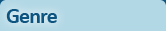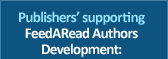# The Little Book of Reversible NumbersReversible numbers are pairs of numbers in which the digits (or numerals) of one number are the reverse of the other, e.g., 39 and 93. The fun starts when the operations of addition, subtraction, multiplication and division are carried out between pairs of such numbers. This book begins with a look at the unexpected way in which addition and subtraction can be carried out. It then looks at how subtraction between reversible pairs leads to an equation that shows the relationship between a number and its reverse, that they are not just two unrelated numbers. Equations for addition and subtraction are derived and are shown to be useful for analysis. Some interesting behaviour occurs when repetitive addition and subtraction are carried out, using the sum or difference as the new number. The book also explores what happens when multiplying and dividing reversible numbers. It is a subject that lends itself to exploration by computer. It is not a book on computing, however. It will appeal to people who enjoy the number patterns that are produced by performing different operations on reversible pairs of numbers. Some useful tools are presented in the appendices, along with web links to various related sites.
ISBN: 9781803022864
Type: Paperback
Pages: 132
Published: 8 December 2021
Price: \$10.25